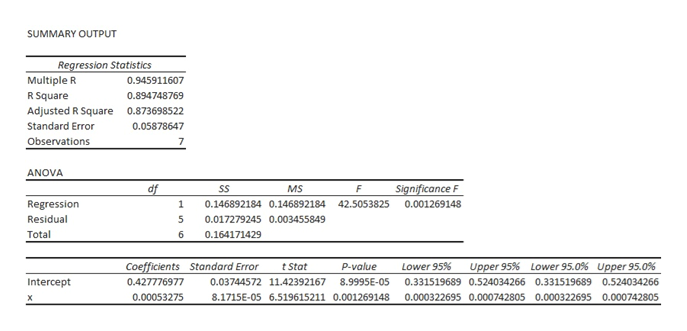# I need help with part (c) only, I need t statistic and p value.Mist (airborne droplets or aerosols) is generated when metal-removing fluids are used in machining operations to cool and lubricate the tool and workpiece. Mist generation is a concern to OSHA, which has recently lowered substantially the workplace standard. An article gave the accompanying data on x = fluid-flow velocity for a 5% soluble oil (cm/sec) and y = the extent of mist droplets having diameters smaller than 10 µm (mg/m3):x88177182354369442970y0.390.600.500.660.610.690.92(a) The investigators performed a simple linear regression analysis to relate the two variables. Does a scatter plot of the data support this strategy? Yes, a scatter plot shows a reasonable linear relationship.No, a scatter plot does not show a reasonable linear relationship.    (b) What proportion of observed variation in mist can be attributed to the simple linear regression relationship between velocity and mist? 0.8947  (c) The investigators were particularly interested in the impact on mist of increasing velocity from 100 to 1000 (a factor of 10 corresponding to the difference between the smallest and largest x values in the sample). When x increases in this way, is there substantial evidence that the true average increase in y is less than 0.6? (Use α = 0.05.) State the appropriate null and alternative hypotheses. H0: β1 = 0.0006667Ha: β1 < 0.0006667 Calculate the test statistic and determine the P-value. (Round your test statistic to two decimal places and your P-value to three decimal places.) t =  P-value =

Question
1 views

I need help with part (c) only, I need t statistic and p value.

Mist (airborne droplets or aerosols) is generated when metal-removing fluids are used in machining operations to cool and lubricate the tool and workpiece. Mist generation is a concern to OSHA, which has recently lowered substantially the workplace standard. An article gave the accompanying data on x = fluid-flow velocity for a 5% soluble oil (cm/sec) and y = the extent of mist droplets having diameters smaller than 10 µm (mg/m3):

 x 88 177 182 354 369 442 970 y 0.39 0.6 0.5 0.66 0.61 0.69 0.92
(a) The investigators performed a simple linear regression analysis to relate the two variables. Does a scatter plot of the data support this strategy?

Yes, a scatter plot shows a reasonable linear relationship.No, a scatter plot does not show a reasonable linear relationship.

(b) What proportion of observed variation in mist can be attributed to the simple linear regression relationship between velocity and mist?

0.8947

(c) The investigators were particularly interested in the impact on mist of increasing velocity from 100 to 1000 (a factor of 10 corresponding to the difference between the smallest and largest x values in the sample). When x increases in this way, is there substantial evidence that the true average increase in y is less than 0.6? (Use α = 0.05.)
State the appropriate null and alternative hypotheses.

H0: β1 = 0.0006667
Ha: β1 < 0.0006667

Calculate the test statistic and determine the P-value. (Round your test statistic to two decimal places and your P-value to three decimal places.)
 t = P-value =

check_circle

Step 1

Part (c):

The regression analysis is conducted here by using EXCEL. The software procedure is given below:

• Enter the data.
• Select Data > Data Analysis > Regression> OK.
• Enter Input Y Range as \$B\$1:\$B\$8.
• Enter Input X Range as \$A\$1:\$A\$8 and click on
• Click OK.

The output using EXCEL is as follows:help_outlineImage TranscriptioncloseSUMMARY OUTPUT Regression Statistics Multiple R 0.945911607 R Square Adjusted R Square 0.894748769 0.873698522 Standard Error 0.05878647 Observations 7 ANOVA Significance F df SS MS F 0.146892184 0.146892184 Regression 1 42.5053825 0.001269148 Residual 0.017279245 0.003455849 Total 6 0.164171429 Upper 95.0 % Coefficients Standard Error Lower 95% Upper 95% Lower 95.0 % tStat P-value Intercept 0.427776977 0.03744572 11.42392167 8.9995E-05 0.331519689 0.524034266 0.331519689 0.524034266 0.00053275 8.1715E-05 6.519615211 0.001269148 0.000322695 0.000742805 0.000322695 0.000742805 x fullscreen
Step 2

The aim of the test is to check whether the true average increase in y is less than 0.6 when x is increased from 100 to 1,000.

The null hypothesis of the form:

H0: β1 = 0.0006667, that is the true average increase in y is 0.6 when x is increased from 100 to 1,000.

The alternative hypothesis is of the form:

H1: β1 < 0.0006667, that is the true average increase in y is less than 0.6 when x is increased from 100 to 1,000.

The test statistic is,

Step 3

Using Excel formula =T.DIST(-1.64, 5,TRUE), the...

### Want to see the full answer?

See Solution

#### Want to see this answer and more?

Solutions are written by subject experts who are available 24/7. Questions are typically answered within 1 hour.*

See Solution
*Response times may vary by subject and question.
Tagged in

### Other